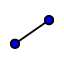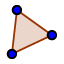Using the Compass Tool: Ex. 3

DIRECTIONS:

﻿You can also use the COMPASS tool to construct an EQUILATERAL TRIANGLE. Here's how: 1) Use the SEGMENTtool to construct a segment with endpoints A and B. 2) Select the COMPASStool. Then select point A, then select point B. (You should notice another circle appear. This circle has a radius = AB). Click on point A. 3) Select the COMPASS tool again. This time, simply click on the segment itself. Then click on point B. 4) Use the INTERSECTtool to plot the point(s) of intersection of the two circles you've just constructed in steps (2) & (3). 5) Use the POLYGONtool to construct the triangle formed by A, B, & the point you constructed in step (4).

6)

Why is this triangle equilateral?

When you're done (or if you're unsure of something), feel free to check by watching the quick silent screencast below the applet.